## Flexible Parametric Representations of Non Parametric Models¶

### 18th March 2015 Neil D. Lawrence¶

#### Inducing point description initially inspired by a notebook of James Hensman¶

First import some relevant libraries and set a random seed.

In :
import GPy
import pods
from GPy.util.linalg import pdinv

from matplotlib import pyplot as plt
%matplotlib inline
import numpy as np

from IPython.display import display

np.random.seed(123)


## Flexible Parametric Approximation to Gaussian Process¶

In this notebook we will try and demonstrate the principles underpinning a flexible parameteric approximation to a non-parameteric model. In particular, the argument goes along these lines. In general, we want to be non-parametric. What do we mean by non-parametric. Here we mean non-parametric in the sense that when we try and represent the relationship between training and test data, $p(\mathbf{y}^\ast|\mathbf{y})$, through a posterior density over a vector of parameters, $\mathbf{w}$, $$p(\mathbf{y}^\ast|\mathbf{y}) = \int p(\mathbf{y}^\ast|\mathbf{w})p(\mathbf{w}|\mathbf{y}) \text{d}\mathbf{w},$$ we find that the vector $\mathbf{w}$ cannot be fixed dimensional.

In a Gaussian process model, we normally relate the observations to a latent function through a likelihood that factorizes, $$p(\mathbf{y}|\mathbf{f}) = \prod_{i=1}^n p(y_i|f_i),$$ Variational inducing variables involve augmenting the prior over functions with a vector of variables, $\mathbf{u}$, $$p(\mathbf{f}) = \int p(\mathbf{f}|\mathbf{u}) p(\mathbf{u}) \text{d}\mathbf{u}$$ and then lower bounding the conditional distribution, $$p(\mathbf{y}|\mathbf{u}) = \int p(\mathbf{y}|\mathbf{f}) p(\mathbf{f}|\mathbf{u}) \text{d}\mathbf{u} \geq \prod_{i=1}^n c_i \hat{p}(\mathbf{y}|\mathbf{u})$$ The lower bound is then used in a model that looks parametric, $$p(\mathbf{y}^*|\mathbf{y}) = \int p(\mathbf{y}^*|\mathbf{u}) \hat{p}(\mathbf{u}|\mathbf{y})\text{d}\mathbf{u},$$ but with the important difference that the number of parameters can be changed at run time not just design time.

## Example¶

### Toy Data Set¶

To show the model working in practice, we are first going to sample a function from a Gaussian process. We will use an exponentiated quadratic covariance function, Sample a data set with two different clusters on the inputs.

In :
N = 50
noise_var = 0.01
X = np.zeros((50, 1))
X[:25, :] = np.linspace(0,3,25)[:,None] # First cluster of inputs/covariates
X[25:, :] = np.linspace(7,10,25)[:,None] # Second cluster of inputs/covariates

# Sample response variables from a Gaussian process with exponentiated quadratic covariance.
k = GPy.kern.RBF(1)
y = np.random.multivariate_normal(np.zeros(N),k.K(X)+np.eye(N)*np.sqrt(noise_var))[:, None]


### Full Gaussian Process¶

Now we have the full data set we will construct a Gaussian process and optimize the parameters, showing the plot of the fit.

In :
m_full = GPy.models.GPRegression(X,y)
m_full.optimize(messages=True) # Optimize parameters of covariance function
_ = m_full.plot() # plot the regression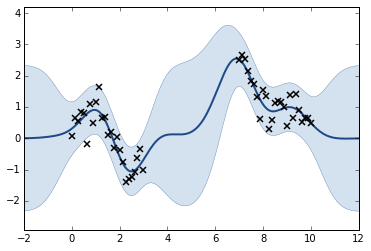### Inducing Variable Approximation¶

In an inducing variable approximation, we introduce 'pseudo-observations' of the function, $\mathbf{u}$, which 'induce' the approximation. We will start by introducing four 'pseudo-observations' at locations 2.5, 4, 7 and 8.5. We will then display the untrained model.

In :
kern = GPy.kern.RBF(1)
Z = np.hstack(
(np.linspace(2.5,4.,2),
np.linspace(7,8.5,2)))[:,None]
m = GPy.models.SparseGPRegression(X,y,kernel=kern,Z=Z)
m.Gaussian_noise.variance = noise_var
m.rbf.variance.constrain_fixed()
m.rbf.lengthscale.constrain_fixed()
m.Gaussian_noise.variance.constrain_fixed()
m.plot()
display(m)

clang: warning: argument unused during compilation: '-fopenmp'
In file included from /Users/neil/.cache/scipy/python27_compiled/sc_1790bf65208b11355ffcfd4b65a5f10913.cpp:11:
In file included from /Users/neil/Library/Enthought/Canopy_64bit/User/lib/python2.7/site-packages/scipy/weave/blitz/blitz/array.h:26:
In file included from /Users/neil/Library/Enthought/Canopy_64bit/User/lib/python2.7/site-packages/scipy/weave/blitz/blitz/array-impl.h:37:
/Users/neil/Library/Enthought/Canopy_64bit/User/lib/python2.7/site-packages/scipy/weave/blitz/blitz/range.h:120:34: warning: '&&' within '||' [-Wlogical-op-parentheses]
return ((first_ < last_) && (stride_ == 1) || (first_ == last_));
~~~~~~~~~~~~~~~~~^~~~~~~~~~~~~~~~~ ~~
/Users/neil/Library/Enthought/Canopy_64bit/User/lib/python2.7/site-packages/scipy/weave/blitz/blitz/range.h:120:34: note: place parentheses around the '&&' expression to silence this warning
return ((first_ < last_) && (stride_ == 1) || (first_ == last_));
^
(                                 )
In file included from /Users/neil/.cache/scipy/python27_compiled/sc_1790bf65208b11355ffcfd4b65a5f10913.cpp:23:
In file included from /Users/neil/Library/Enthought/Canopy_64bit/User/lib/python2.7/site-packages/numpy/core/include/numpy/arrayobject.h:4:
In file included from /Users/neil/Library/Enthought/Canopy_64bit/User/lib/python2.7/site-packages/numpy/core/include/numpy/ndarrayobject.h:17:
In file included from /Users/neil/Library/Enthought/Canopy_64bit/User/lib/python2.7/site-packages/numpy/core/include/numpy/ndarraytypes.h:1761:
/Users/neil/Library/Enthought/Canopy_64bit/User/lib/python2.7/site-packages/numpy/core/include/numpy/npy_1_7_deprecated_api.h:15:2: warning: "Using deprecated NumPy API, disable it by "          "#defining NPY_NO_DEPRECATED_API NPY_1_7_API_VERSION" [-W#warnings]
#warning "Using deprecated NumPy API, disable it by " \
^
#include <omp.h>
^
2 warnings and 1 error generated.

Weave compilation failed. Falling back to (slower) numpy implementation

WARNING: reconstraining parameters sparse_gp_mpi.rbf.variance
WARNING: reconstraining parameters sparse_gp_mpi.rbf.lengthscale
WARNING: reconstraining parameters sparse_gp_mpi.Gaussian_noise.variance


Model: sparse gp mpi
Log-likelihood: -1822.27351085
Number of Parameters: 7

sparse_gp_mpi. Value Constraint Prior Tied to
inducing inputs (4, 1)
rbf.variance 1.0 fixed
rbf.lengthscale 1.0 fixed
Gaussian_noise.variance 0.01 fixed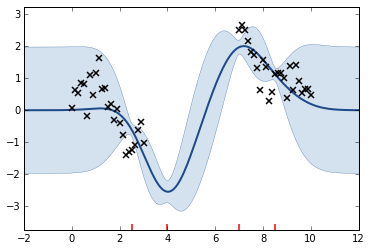When the prior over $\mathbf{u}$ is integrated over the effective likelihood, $\hat{p}(\mathbf{y}|\mathbf{u})$, it's possible to show that the resulting marginal likelihood, $\hat{p}(\mathbf{y})$, which forms the core of the lower bound on the likelihood, is a Gaussian process with a low rank form for the covariance, $$\hat{p}(\mathbf{y}) \sim \mathcal{N}(\mathbf{0}, \hat{\mathbf{K}})$$ where $$\hat{\mathbf{K}} = \mathbf{K}_{fu}\mathbf{K}_{uu}^{-1} \mathbf{K}_{uf} + \sigma^2 \mathbf{I},$$ where $\mathbf{K}_{fu}$ gives the cross covariance between the variables of our function $f()$ and those of the inducing functions $u()$.

Let's have a look at the form of this approximation for the un-optimized inducing variables.

In :
# Visualise full covariance and approximation.
Kff = m_full.kern.K(m.X,m.X)
Kfu = m.kern.K(m.X, m.Z)
Kuu = m.kern.K(m.Z, m.Z)
Kuf = Kfu.T
noise = m['.*noise']
fig, ax = plt.subplots(1, 2, figsize=(12, 8))
ax.matshow(np.dot(np.dot(Kfu,pdinv(Kuu)),Kuf)  + np.eye(m.X.shape)*m['.*noise'])
ax.set_title('Low Rank Approximation')
ax.matshow(Kff + np.eye(m.X.shape)*m['.*noise'])
ax.set_title('Full Covariance')

Out:
<matplotlib.text.Text at 0x110234250>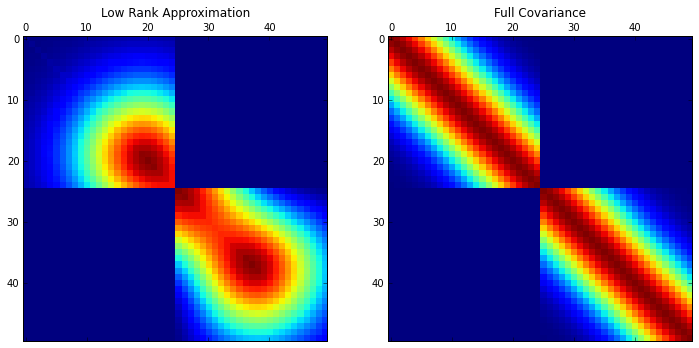### Variational Compression¶

The variational compression bound minmizes the additional information obtained about $\mathbf{f}$ by knowing $\mathbf{y}$ with respect to that which is known by observing $\mathbf{u}$ alone. Maximizing the lower bound (whilst keeping model parameters fixed) compresses the information from $\mathbf{y}$ into $q(\mathbf{u})$.

In :
m.optimize(messages=True)
m.plot()
display(m)


Model: sparse gp mpi
Log-likelihood: -832.753958521
Number of Parameters: 7

sparse_gp_mpi. Value Constraint Prior Tied to
inducing inputs (4, 1)
rbf.variance 1.0 fixed
rbf.lengthscale 1.0 fixed
Gaussian_noise.variance 0.01 fixed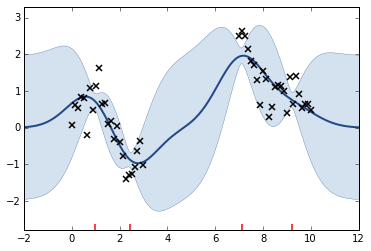In :
m.randomize()
m.Z.unconstrain()
m.optimize('bfgs', messages=True)
_ = m.plot()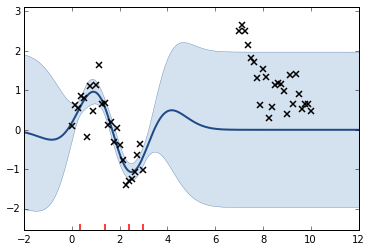### Joint Optimization¶

In practice we actually optimize the model parameters alongside the variational parameters. This means we either find better solutions, or solutions where it's easier to compress the information from $\mathbf{y}$ into $\mathbf{u}$. The former case occurs because for certain choices of prior over $\mathbf{f}$ the values of $\mathbf{y}$ do not provide a lot of information.

In :
M = 8
Z = np.random.rand(M,1)*12
m = GPy.models.SparseGPRegression(X,y,kernel=kern,Z=Z)
m.likelihood.variance = noise_var

fig, ax = plt.subplots(1, 2, figsize=(16, 8))
m.optimize(messages=True)
m.plot(ax=ax)
m_full.plot(ax=ax)
print M, "inducing variables"
print "Full model log likelihood: ", m_full.log_likelihood()
print "Lower bound from variational method: ", m.log_likelihood()
print "Information gain (in nats) associated with y ", m_full.log_likelihood() - m.log_likelihood()

8 inducing variables
Full model log likelihood:  -36.4462976038
Lower bound from variational method:  [[-39.99802542]]
Information gain (in nats) associated with y  [[ 3.55172782]]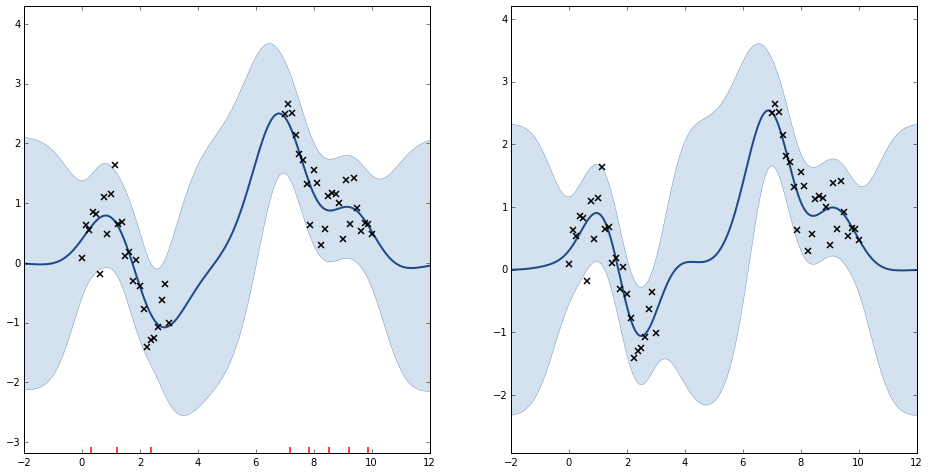In :
Kff = m_full.kern.K(m.X,m.X)
Kfu = m.kern.K(m.X, m.Z)
Kuu = m.kern.K(m.Z, m.Z)
Kuf = Kfu.T
sigma2 = m.likelihood.variance
KfuKuuIKuf = np.dot(np.dot(Kfu,pdinv(Kuu)),Kuf)
fig, ax = plt.subplots(1, 2, figsize=(12, 6))
ax.matshow(KfuKuuIKuf  + np.eye(m.X.shape)*sigma2)
ax.set_title('Low Rank Approximation')
ax.matshow(Kff + np.eye(m.X.shape)*sigma2)
_ = ax.set_title('Full Covariance')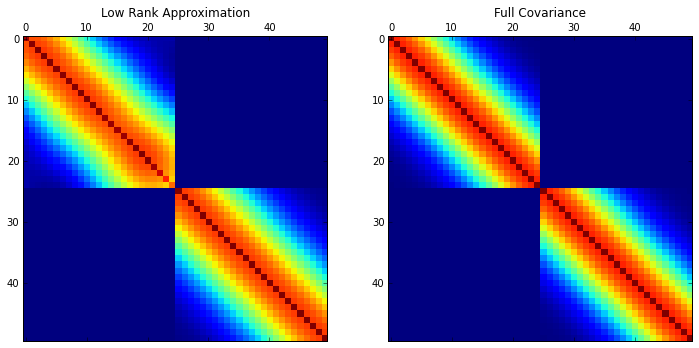## Ceres Data¶

Now we will look at some real data. This is data as published by Franz von Zach's volume, Monatliche Correspondenz by Giuseppe Piazzi for observations of the (dwarf) planet Ceres, as shown in the Google book below. }

In :
pods.notebook.display_google_book('JBw4AAAAMAAJ', 'PA280')


We've made the data available in the pods library. The data is famous because Gauss fitted the orbit of Ceres, and made a made a prediction about the location of the planet a year after its discovery, allowing the planet to be recovered (it had been lost behind the sun). This established Gauss at the age of 23 as a leading European mathematician. Gauss later claimed that he used the Gaussian error model when making his predictions. The data is in the form of a pandas data frame, which can be loaded and summarized as follows.

In :
import pods
data = pods.datasets.ceres()['data']
data.describe()

Out:
Mittlere Sonnenzeit Gerade Aufstig in Zeit Gerade Aufstiegung in Graden Nordlich Abweich Geocentrische Laenger Geocentrische Breite Ort der Sonne + 20" Aberration Logar. d. Distanz
count 21.000000 21.000000 21.000000 20.000000 19.000000 19.000000 19.000000 19.000000
mean 7.460553 3.474579 52.117897 17.052194 1.867660 1.802494 10.089094 9.993320
std 0.784663 0.055710 0.836052 0.985692 0.036103 0.815463 0.444599 0.000609
min 6.199500 3.424925 51.374056 15.628750 1.832791 0.600806 9.396909 9.992616
25% 6.807333 3.435597 51.533972 16.329201 1.839015 1.153542 9.780756 9.992807
50% 7.400750 3.448292 51.724389 17.015889 1.851418 1.707806 10.084920 9.993189
75% 8.038194 3.504792 52.571889 17.827847 1.889333 2.383375 10.431290 9.993735
max 8.721611 3.618483 54.277250 18.799667 1.952000 3.111694 10.813114 9.994582

## Gaussian Process Fit to Ceres Data¶

Now let's try fitting 'Gerade Aufstig in Zeit' using a Gaussian process. First thing to do is remove the missing value.

In :
X = np.delete(np.asarray(data.index.dayofyear, dtype='float')[:, None], 8, axis=0)
y = np.delete(np.asarray(data['Gerade Aufstig in Zeit'])[:, None], 8, axis=0)


Now we will use an exponentiated quadratic covariance. Because observations are in days, we initialise the lengthscale to 10 days. Also the noise on these observations turns out to be very low. To prevent numerical problems, we add a bound to prevent the noise going below 1e-6.

In :
kern = GPy.kern.RBF(1)
kern.lengthscale = 10.

m = GPy.models.GPRegression(X, y, kern)
# noise is so low that we get numerical issues if we allow noise variance to be 'free'
m.Gaussian_noise.variance.constrain_bounded(1e-6, 10)
m.optimize(messages=True)
m.plot()
display(m)

WARNING: reconstraining parameters GP_regression.Gaussian_noise.variance


Model: GP regression
Log-likelihood: 99.4357991358
Number of Parameters: 3

GP_regression. Value Constraint Prior Tied to
rbf.variance 24.1179178618 +ve
rbf.lengthscale 141.255644761 +ve
Gaussian_noise.variance 1e-061e-06,10.0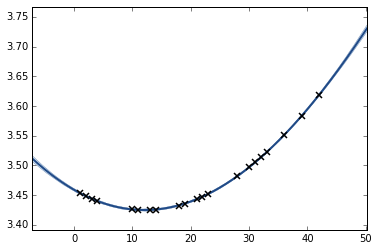Note the log likelihood of the fit which is just over 99.43.

## Low Rank Gaussian Process Fit¶

Now we form the same fit again but using a low rank Gaussian process. Buy changing the number of inducing variables we capture different aspects of the function. We'll start with one inducing variable. What aspect of the function do we expect to capture if we only store 1 thing about it? For each fit check the log likelihood of the result and compare it to the log likelihood of the full Gaussian process above (it should always lower bound this value). How many inducing variables do we need to capture this sequence?

In :
M = 4
Z = np.random.rand(M,1)*45
kern2 = GPy.kern.RBF(1)
kern2.lengthscale = 10.
m2 = GPy.models.SparseGPRegression(X, y, kern2, Z=Z)
m2.Gaussian_noise.variance.constrain_bounded(1e-6, 10)
m2.optimize(messages=True)
_ = m2.plot()

WARNING: reconstraining parameters sparse_gp_mpi.Gaussian_noise.variance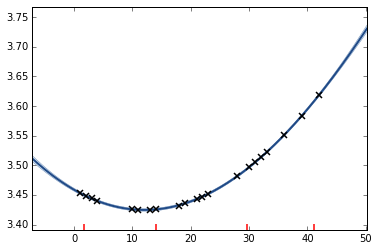## Some Things to Try¶

Do you always get the same result for different initialisations of the inputs to the inducing variables? Can you recover the full model likelihood for enough inducing inputs? If not, why is that?